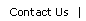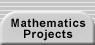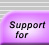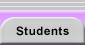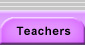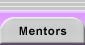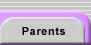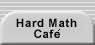# Modular Arithmetic

Modular arithmetic is based on a number's remainder after division by a divisor (called the modulus).

For an explanation and exercises, see Modular Arithmetic, Clock Arithmetic, and More Clock Arithmetic.

Further discussion and activities can be found at Clock Arithmetic Pages (the Number Bracelet activity makes for an interesting research investigation).

For a more technical discussion, see Modular Arithmetic and its interactive modular arithmetic table. Notation note: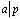means that a divides p (and implies that p is congruent to 0 (mod a)).Translations of mathematical formulas for web display were created by tex4ht. © Copyright 2003 Education Development Center, Inc. (EDC)